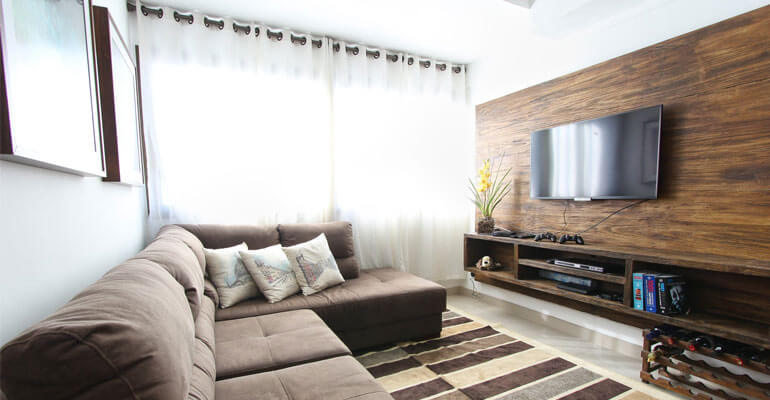Of all the elements that make a room perfect and beautiful, lighting is perhaps the most important one. Whatever be the type of décor you adopt, the items you hang on the wall and the colour you paint them with, if the lighting is dull and not complementing the room, all your efforts that went to make the room look the way it looks now, will go waste. This is why you need to understand the importance of calculating the light needed for each of your rooms depending on various elements.Let us know to try to understand the factors to be considered while selecting appropriate lighting for the room.

#### The location of the room:

As you might understand, the lighting required for a room differed according to the location of the room. If the room falls either on the sunset or sundown side would normally require lesser light during the morning or in the evening. However, if the room falls deeper into the house where there is no natural lighting, the amount of light needed in the room will be higher.

#### The décor of the room:

Depending on the décor of the room, the amount of light needed by the room would also vary. A room decorated with items with less reflective capacity, naturally, the amount of lighting needed would be more as these items do not reflect light but only absorb the light making the room look dull. On the other side, if the items have brighter shades that reflect light, the amount of light required is normally less.

#### The colour of the paint:

This goes with the previous point that we have discussed. Paints that fall towards the white end of the colour spectrum—or lighter colours—have the ability to make the room look brighter even when in lower light. However, on the flipside, if the room makes use of dark colors, naturally it tends to absorb light making the room dull.

Now, let us see how to calculate the amount of light needed based on the size of the room.

The amount of light required is calculated in either Lux or Lumen, where Lux denotes the amount of light per square meter and Lumen represents the light intensity. It has to be understood that most of the lights mention their lumens they can produce in their description. Hence, all you need to calculate is the Lumens you require and then buying a light that can produce that much Lumen.

In order to calculate the area of a room, you need to get the following details of the same;

Now, Area= Length X Breadth Square Meters

If you are measuring the room in square feet, multiply the Area by 0.093 to get the area in square meters.

How much is needed by each room?

Depending upon the standard conditions, the light requirements of each room varies. Let us see, how much light is needed for each room.

• Home Office: About 500 lx
• Dining Room: About 200 lx

Now, to get the lumens required for a bedroom, multiply the lx of the room with the area.

For example,

For a room with 10 feet length and 5 feet breadth;

Area= 10×5 = 50 sq.feet

In meters; 50 sq.ft. X 0.093 = 4.65 sq. meters.

Now, to get the lumens;

4.65 sq. meters x 300 = 1395 Lumens.

So, the lumens required by a bedroom with the above area are 1395 lumens. Likewise, you can calculate the lumens required by any room.